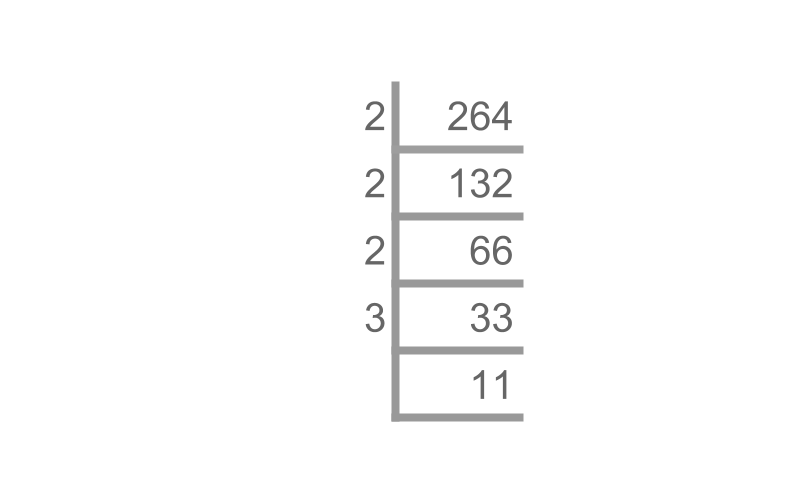maths > wholedivisors

Factors, Multiples, Prime Factorization

what you'll learn...

overview

This page provides a brief overview of factors of a number. multiplies of a number. prime factorization of numbers

Factors

When the remainder is 0$0$, a dividend is "divisible" by a divisor.

The divisors that divide, with remainder 0$0$, are called factors of the dividend.

eg: Factors of 6$6$ are 1$1$, 2$2$, 3$3$, and $6$. Because the remainder is $0$ for $6 \div 1$, $6 \div 2$, $6 \div 3$ and $6 \div 6$

eg: Factors of $20$ are $1$, $2$, $4$, $5$, $10$, and $20$

eg: Factors of $13$ are $1$ and $13$

The word "factor" means "a part or component of something bigger".

Factors of a number : The divisor that divides with remainder $0$, is a factor of the dividend.

the dividend, divisor are non-zero whole numbers.

The factors of $26$ are "$1 , 2 , 13 , 26$".

The factors of $28$? are "$1 , 2 , 4 , 7 , 14 , 28$".

Multiples

Consider the number $6$. The following shows result of multiplying $6$ by the sequence of numbers $1 , 2 , 3 , 4 , 5 , \cdots$
"$6$, $12$, $18$, $24$, $30$, $\cdots$ "

A dividend that has another number as factor is called multiple of the factor.

eg: $24$ is a multiple of $3$, as $24 \div 3 = 8$ with remainder $0$

eg: $24$ is also a multiple of $2$

eg: $24$ is also a multiple of $6$

eg: $24$ is also a multiple of $24$

The word "multiple" means "having several parts of something".

Multiples of a number : The dividend that is divided by a factor with remainder $0$, is a multiple of the factor.

The multiples of $6$ are "$6 , 12 , 18 , \cdots$".

The multiples of $34$ are "$34 , 68 , 102 , 136 , \cdots$".

Prime Factorization

The factors of $24$ are "$1 , 2 , 3 , 4 , 6 , 8 , 12 , 24$"

Consider the number $24$. Factors of $24$ are $1$, $2$, $3$, $4$, $6$, $8$, $12$, and $24$. The following ways, the number is expressed as a product of some of the factors.
$4 \times 6$
$2 \times 4 \times 3$
$3 \times 8$
and more like $2 \times 12$

The following is a product of prime numbers
"$2 \times 2 \times 2 \times 3$". The number $24$ is represented as product of prime factors. This is called prime factorization.

Prime Factorization : A number represented as product of prime numbers.What is the prime factorization of $264$?
The answer is "product of $2 \times 2 \times 2 \times 3 \times 11$".
A procedure is illustrated in the figure.

What is the prime factorization of $17$?
Since $17$ is a prime number, the answer is "$17$".

summary

Factors of a number : The divisor that divides with remainder $0$, is a factor of the dividend.

the dividend, divisor are non-zero whole numbers.

Multiples of a number : The dividend that is divided by a factor with remainder $0$, is a multiple of the factor.

Prime Factorization : A number represented as product of prime numbers.

Outline

The outline of material to learn "Divisibility in Whole Numbers" is as follows.

→   Classification as odd, even, prime, and composite

→   Factors, Multiples, Prime factorization

→   Highest Common Factor

→   Lowest Common Multiple

→   Introduction to divisibility tests

→   Simple Divisibility Tests

→   Simplification of Divisibility Tests

→   Simplification in Digits for Divisibility Tests